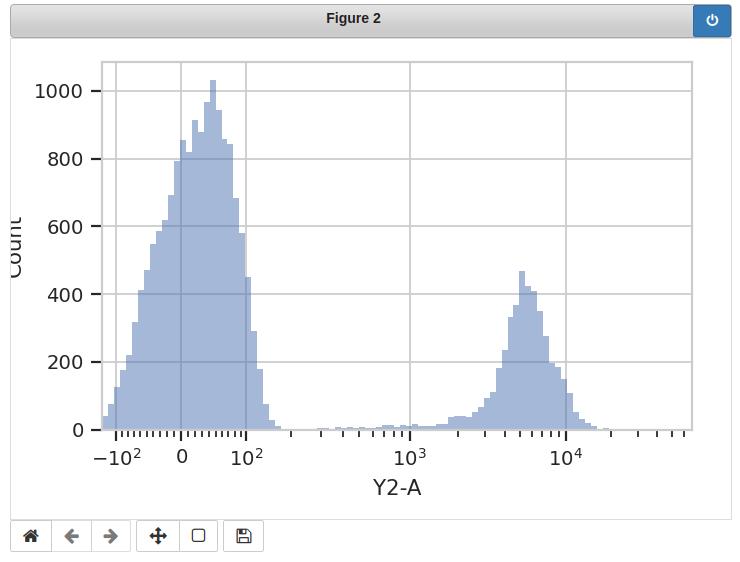These are some questions that are commonly asked about Cytoflow’s modules, especially by new users.

Cytoflow seems like it could be useful for analyzing data other than FCS files. Can I do that?

Maybe! Quite a few portions of the package assume that the data is from a flow cytometer. This is quite explicitly baked into the point-and-click GUI; less so with the Python modules. If you want to give it a go (and are using the Python modules), have a look at the `Experiment` class. You’ll have to build one of those manually – instead of creating one with `ImportOp` – and you’ll obviously need to avoid modules that explicitly expect other FCS files to parameterize them. But otherwise, once you’ve got a fully-formed Experiment, you should (probably) be fine to use (most of) the rest of the package(?) Let me know how you get on.

How do I save the plots I’ve made with the Python modules?

`cytoflow` uses the `matplotlib.pyplot` stateful interface for making plots. Thus, there are two ways to save the plots. The first is to use the `matplotlib.pyplot.savefig()` – see the `matplotlib` documentation for more details. (This option gives you the greatest flexibility in format, resolution, etc.)

If you are using the Jupyter notebook, the second is to replace the `%matplotlib inline` magic with `%matplotlib notebook`. Then, when you make a plot, the plot remains interactive. Here’s an example: